# Predator prey

Jump to: navigation, search

## Contents

This page contains a description predator prey model that is used as a running example throughout the text. A detailed description of the dynamics of this system is presented in Chapter 3 - Examples and these dynamics are analyzed in Chapter 4 - Dynamic Behavior. A state space feedback controller is designed in Chapter 6 - State Feedback. This page brings together this material into a single place, to illustrate the application of analysis and design tools for this system. Links to MATLAB scripts are included that generate the analysis and figures described here.

### System Description

The predator-prey problem refers to an ecological system in which we have two species, one of which feeds on the other. This type of system has been studied for decades and is known to exhibit interesting dynamics. The figure below shows a historical record taken over 90 years for a population of lynxes versus a population of hares (MacLulich, 1937).Figure 2.6: Predator versus prey. The photograph on the left shows a Canadian lynx and a snowshoe hare, the lynx’s primary prey. The graph on the right shows the populations of hares and lynxes between 1845 and 1935 in a section of the Canadian Rockies (MacLuluch, 1937). The data were collected on an annual basis over a period of 90 years. (Photograph copyright Tom and Pat Leeson.)

#### Discrete Time Model

A simple model for this situation can be constructed using a discrete-time model by keeping track of the rate of births and deaths of each species. Letting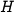represent the population of hares and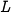represent the population of lynxes, we can describe the state in terms of the populations at discrete periods of time. Lettingbe the discrete-time index (e.g., the day or month number), we can write

whereis the hare birth rate per unit period and as a function of the food supply,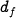is the lynx mortality rate andandare the interaction coefficients. The interaction term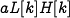models the rate of predation, which is assumed to be proportional to the rate at which predators and prey meet and is hence given by the product of the population sizes. The interaction term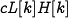in the lynx dynamics has a similar form and represents the rate of growth of the lynx population. This model makes many simplifying assumptions -- such as the fact that hares decrease in number only through predation by lynxes -- but it often is sufficient to answer basic questions about the system.

To illustrate the use of this system, we can compute the number of lynxes and hares at each time point from some initial population. This is done by starting with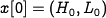and then using equation (2.13) to compute the populations in the following period. By iterating this procedure, we can generate the population over time. The output of this process for a specific choice of parameters and initial conditions is shown below:

Using the parameters,andin equation~\eqref{eq:modeling:predprey} with daily updates, the period and magnitude of the lynx and hare population cycles approximately match the data in Figure 2.4. While the details of the simulation are different from the experimental data (to be expected given the simplicity of our assumptions), we see qualitatively similar trends and hence we can use the model to help explore the dynamics of the system.

MATLAB files for the discrete time model:

#### Continuous Time Model

We now replace the difference equation model used there with a more sophisticated differential equation model. Let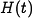represent the number of hares (prey) and let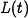represent the number of lynxes (predator). The dynamics of the system are modeled as

In the first equation,represents the growth rate of the hares,represents the maximum population of the hares (in the absence of lynxes),\index{carrying capacity, in population models}represents the interaction term that describes how the hares are diminished as a function of the lynx population andcontrols the prey consumption rate for low hare population. In the second equation,represents the growth coefficient of the lynxes and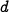represents the mortality rate of the lynxes. Note that the hare dynamics include a term that resembles the logistic growth model (3.30) in Chapter 3 - Examples.

Of particular interest are the values at which the population values remain constant, called equilibrium points. The equilibrium points for this system can be determined by setting the right-hand side of the above equations to zero. Lettingandrepresent the equilibrium state, from the second equation we have

Thus, we have three possible equilibrium points:where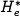andare given in equations (3.32) and (3.33). Note that the equilibrium populations may be negative for some parameter values, corresponding to a nonachievable equilibrium point.

Figure 3.20 shows a simulation of the dynamics starting from a set of population values near the nonzero equilibrium values.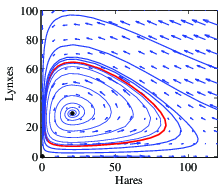Figure 3.20: Simulation of the predator-prey system. The figure on the left shows a simulation of the two populations as a function of time. The figure on the right shows the populations plotted against each other, starting from different values of the population. The oscillation seen in both figures is an example of a limit cycle. The parameter values used for the simulations are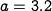,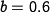,,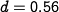,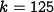and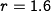.

We see that for this choice of parameters, the simulation predicts an oscillatory population count for each species, reminiscent of the data shown in Figure 2.6.

MATLAB files:

• predprey_dynamics.m - MATLAB code to generate the simulation and phase portrait
• predprey.m - system definition (for use by ode45 and amphaseplot)

See the software page for more information on how to run these scripts.

### Dynamic Behavior

To assess the stability of the equilibrium point, we evaluate the linearization of the dynamics about the point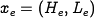. A tedious but otherwise straightforward computation gives that the dynamics matrix for the linearized system is given by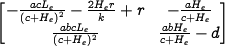The stability of the system can be computed by evaluating eigenvalues of this matrix at different equilibrium points.

To explore how the parameters of the model affect the behavior of the system, we choose to focus on two specific parameters of interest:, the interaction coefficient between the populations and, a parameter affecting the prey consumption rate. Figure 4.17a is a numerically computed {\em ^{parametric stability diagram}} showing the regions in the chosen parameter space for which the equilibrium point is stable (leaving the other parameters at their nominal values).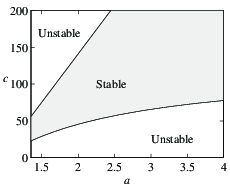(a) Stability diagram (b) Bifurcation diagram Figure 4.17: Bifurcation analysis of the predator-prey system. (a) Parametric stability diagram showing the regions in parameter space for which the system is stable. (b) Bifurcation diagram showing the location and stability of the equilibrium point as a function of. The solid line represents a stable equilibrium point, and the dashed line represents an unstable equilibrium point. The dashed-dotted lines indicate the upper and lower bounds for the limit cycle at that parameter value (computed via simulation). The nominal values of the parameters in the model are,,,,and.

We see from this figure that for certain combinations ofandwe get a stable equilibrium point, while at other values this equilibrium point is unstable.

Figure 4.17b is a numerically computed bifurcation diagram for the system. In this plot, we choose one parameter to vary () and then plot the equilibrium value of one of the states () on the vertical axis. The remaining parameters are set to their nominal values. A solid line indicates that the equilibrium point is stable; a dashed line indicates that the equilibrium point is unstable. Note that the stability in the bifurcation diagram matches that in the parametric stability diagram for(the nominal value) andvarying from 1.35 to 4. For the predator-prey system, when the equilibrium point is unstable, the solution converges to a stable limit cycle. The amplitude of this limit cycle is shown by the dashed-dotted line in Figure 4.17b.

MATLAB and Mathematica files:

• predprey_bif.mma - Mathematica file for doing symbolic calculations on the model
• predprey.mma - Mathematica file for doing symbolic calculations on the model
• Jac.m - Mathematica file computing the Jacobian of a vector field
• predprey_bif.m - MATLAB code to generate the bifurcation diagram

See the software page for more information on how to run these scripts.

### State Feedback

Consider the problem of regulating the population of an ecosystem\index{ecosystems} by modulating the food supply. We use the predator--prey model introduced in Section Template:Sec:examples:predprey. The dynamics for the system are given by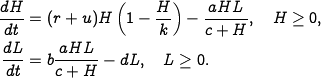We choose the following nominal parameters for the system, which correspond to the values used in previous simulations: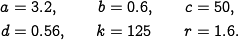We take the parameter, corresponding to the growth rate for hares, as the input to the system, which we might modulate by controlling a food source for the hares. This is reflected in our model by the termin the first equation. We choose the number of lynxes as the output of our system.

To control this system, we first linearize the system around the equilibrium point of the system, which can be determined numerically to be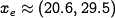. This yields a linear dynamical systemwhere,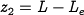and. It is easy to check that the system is reachable around the equilibrium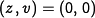, and hence we can assign the eigenvalues of the system using state feedback.

Determining the eigenvalues of the closed loop system requires balancing the ability to modulate the input against the natural dynamics of the system. This can be done by the process of trial and error or by using some of the more systematic techniques discussed in the remainder of the text. For now, we simply choose the desired closed loop eigenvalues to be at. We can then solve for the feedback gains using the techniques described earlier, which results in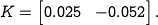Finally, we solve for the reference gain, using equation~\eqref{eq:statefbk:kr} to obtain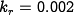.

Putting these steps together, our control law becomeswhere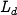is the desired number of lynxes. In order to implement the control law, we must rewrite it using the original coordinates for the system, yieldingThis rule tells us how much we should modulateas a function of the current number of lynxes and hares in the ecosystem. Figure 6.7a shows a simulation of the resulting closed loop system using the parameters defined above and starting with an initial population of 15 hares and 20 lynxes.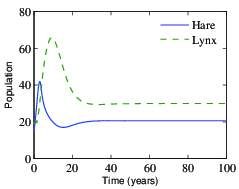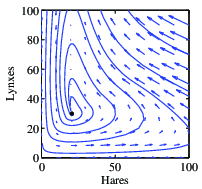(a) Initial condition response (b) Phase portrait Figure 6.7: Simulation results for the controlled predator--prey system. The population of lynxes and hares as a function of time is shown in (a), and a phase portrait for the controlled system is shown in (b). Feedback is used to make the population stable at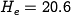and.

Note that the system quickly stabilizes the population of lynxes at the reference value (). A phase portrait of the system is given in Figure 6.7b, showing how other initial conditions converge to the stabilized equilibrium population. Notice that the dynamics are very different from the natural dynamics (shown in Figure 3.20).

MATLAB files:

See the software page for more information on how to run these scripts.